Rush Hour?

Rush Hour?
bluenemo 發表於 2013-11-24 14:47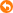n-number puzzle, but with two or more slots, also number repeated
(start)-->(goal)
323         233
405          450
067 -->  670
something like that

i don't know how to solve this...... i know there have IDA* concept but algorithm hard to think....T-T

bluenemo 發表於 2013-11-24 15:15i have some idea, but cannot cover all cases, i want someone can guide me how to do....
my first think is, find the shortest path between each number, when shortest path found, other number not link this number for shortest path
for example
120        220
320 --> 130
000         000
shortest path --> 1->1(cost:1), 2->2(cost:0), 2->2(cost:2), 3-->3(cost:1), total cost = 4
aleast 4 step to come to goal
second think is, start from slot (0), test move if each slot have the number in 4 position: up, down, right, left. if swaped, check the cost again to find the best path. for each iteration, base cost will increase 1.
but the problem is....some cases cannot fulfill the above algorithm, for example
120        020
322 --> 322
000         001
i don't know how to solve this case.......can give me a some hints?

Not quite sure if i got your game correct... is this like this:

http://en.wikipedia.org/wiki/15_puzzle

Not quite sure if i got your game correct... is this like this:
bluenemo 發表於 2013-11-24 15:37thanks, but.....not useful

e.g.
123
321
120

123
321
012

LL

e.g.
123
245
670
final:
122
345
670

Check if you can formulate your solution in this form:

http://www.cs.sjsu.edu/~stamp/cv/papers/rh.pdf

If yes, either Dijkstra's algorithm or IDA* search will be able to help search the solution.

IF right pattern -> true
DONE

IF right pattern -> true
DONE

Jimmy0911 發表於 2013-11-24 21:20Jimmy0911 發表於 2013-11-25 00:23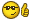year one project未去到ai

btw....





1係2，2都可以係2
background 處理用唔重覆既number

checking

btw....





Jimmy0911 發表於 2013-11-25 01:10e.g.
1203
3040
4460
1456
final:
4002
3010
4466
1453

Jimmy0911 發表於 25/11/2013 09:04 AM呢個係o(n^2) ge問題

The n-puzzle is a classical problem for modelling algorithms involving heuristics. Commonly used heuristics for this problem include counting the number of misplaced tiles and finding the sum of the Manhattan distances between each block and its position in the goal configuration. Note that both are admissible, i.e., they never overestimate the number of moves left, which ensures optimality for certain search algorithms such as A*.

The n-puzzle is a classical problem for modelling algorithms involving heuristics. Commonly used heu ...
Jackass_TMxCK 發表於 2013-11-25 18:59PM

oh,sor

IF right pattern -> true
DONE

Jimmy0911 發表於 2013-11-24 21:20How do you check all the configurations of various moves?
Do you know that there are an exponential number of states?
How can you make sure your program run fast enough?

Chess without AI is merely hard-coding.
This problem is more challenging.

Here is the pseudocode of the basic algorithm

IDAStar(State s, int limit) {
mark state s as visited
if s is ending state {
print the path by tracing the predecessor of s
} else {
for all neighbour state s' {
if s' is not visited && limit >= cost of s' + h(s') {
mark s' as visited
record s as the predecessor of s'
IDAStar(s', limit)
}
}
}
}

where h(s') is an admissible heuristic function, for example, sum of manhattan distances of each tiles.

Here is the pseudocode of the basic algorithm

IDAStar(State s, int limit) {
mark state s as vis ...
KoolFreeze 發表於 2013-11-26 01:16IDAStar(s', limit - (cost of s' + h(s')))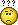why limit - (cost of s' + h(s'))
also, this algorithm not consider more than 1 slot and duplicate number
but as you said, just basic algorithm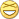My mistake... No need to update the limit.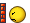Just keep calling IDAStar with an increasing limit until a solution can be found.

also, this algorithm not consider more than 1 slot and duplicate number

It just depends on how you enumerate neighbour states.

It just depends on how you enumerate neighbour states.
KoolFreeze 發表於 2013-11-26 01:48as i said....not easy to guess since duplicate number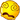A simple solution is to find all spaces and swap each with any adjacent tile.

For heuristic function, you don't need to insist on matching each tile of the same digit to a different location. Otherwise, it becomes a Maximum Weight Matching problem, which can be quite complicated. You can simply use the shortest manhattan distance to that digit at the target state. It is still an admissible heuristic.

I've written an example program. It can handle arbitrary number of slots and duplicate numbers. I only focus on simplicity rather than speed, so there are still many rooms for optimization.
1. #include <iostream>
2. #include <vector>
3. #include <map>

4. using namespace std;

5. typedef vector< vector<int> > State;

6. map<State, bool> visited;
7. vector<State> path;
8. State start, target;
9. int N;

10. void input(State &s) {
11.   s = State(N, vector<int>(N));
12.   for (int i = 0; i < N; ++i) for (int j = 0; j < N; ++j) cin >> s[i][j];
13. }

14. inline int h(const State &s, const State &t) {
15.   int a, b, c, d, tot = 0;
16.   for (a = 0; a < N; ++a) for (b = 0; b < N; ++b) if (s[a][b] != 0) {
17.     int best = N + N;
18.     for (c = 0; c < N; ++c) for (d = 0; d < N; ++d) if (t[c][d] == s[a][b]) {
19.       if (best > abs(a - c) + abs(b - d)) best = abs(a - c) + abs(b - d);
20.     }
21.     tot += best;
22.   }
24. }

25. bool IDAStar(const State &s, int cost, int limit) {
26.   visited[s] = true;
27.   path.push_back(s);
28.   if (s == target) {
29.     for (int x = 0; x <= cost; ++x) {
30.       cout << "== Step " << x << " ==" << endl;
31.       for (int i = 0; i < N; ++i) {
32.         for (int j = 0; j < N; ++j) cout << path[x][i][j] << ' ';
33.         cout << endl;
34.       }
35.     }
36.   } else if (cost + h(s, target) <= limit) {
37.     for (int i = 0; i < N; ++i) for (int j = 0; j < N; ++j) if (s[i][j] == 0) {
38.       for (int d = 0; d < 4; ++d) {
39.         int ni = i + ((d + 1) % 2) * (d - 1), nj = j + (d % 2) * (d - 2);
40.         if (ni >= 0 && ni < N && nj >= 0 && nj < N && s[ni][nj] != 0) {
41.           State ns(s);
42.           swap(ns[i][j], ns[ni][nj]);
43.           if (!visited[ns] && IDAStar(ns, cost + 1, limit)) return true;
44.         }
45.       }
46.     }
47.   }
48.   path.pop_back();
49.   return s == target;
50. }

51. int main() {
52.   cout << "N? ";
53.   cin >> N;
54.   cout << "Starting state?" << endl;
55.   input(start);
56.   cout << "Target state?" << endl;
57.   input(target);
58.   for (int limit = 0; !IDAStar(start, 0, limit); ++limit) {
59.     visited.clear();
60.     cout << "Searching with limit " << limit << "..." << endl;
61.   }
62.   return 0;
63. }

Example input
1. N? 4
2. Starting state?
3. 1 2 0 3
4. 3 0 4 0
5. 4 4 6 0
6. 1 4 5 6
7. Ending state?
8. 4 0 0 2
9. 3 0 1 0
10. 4 4 6 6
11. 1 4 5 3

I've written an example program. It can handle arbitrary number of slots and duplicate numbers. I on ...
KoolFreeze 發表於 2013-11-27 16:31Sorry, your algorithm cannot pass the following case
5
50204
34030
63310
35361
23111

5
30002
34050
63311
35364
23111

So that what i said cannot cover all of cases......

but anyway, thanks for your help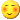i think the key point is, h will changed in every state, and due to duplicate number, h are not accurate
may need to use dynamic programmingi think the key point is, h will changed in every state, and due to duplicate number, h are not accu ...

dynamic programming is to optimize a solution rather than a solution itself?

delete.

anyone can helpI personally would suggest you consult your lecturer for your course work problem, you paid for that, and thats how you learn from the course, if all you do is coming to a public forum and cry for help, why don't you just learn yourself instead of going for the course?